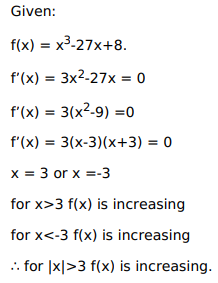# Solve this following

Question:

Mark $(\sqrt{)}$ against the correct answer in the following:

The function $f(\mathrm{x})=\mathrm{x}^{3}-27 \mathrm{x}+8$ is increasing when

A. $|\mathrm{x}|<3$

B. $|\mathrm{x}|>3$

C. \$-3

D. none of these

Solution: